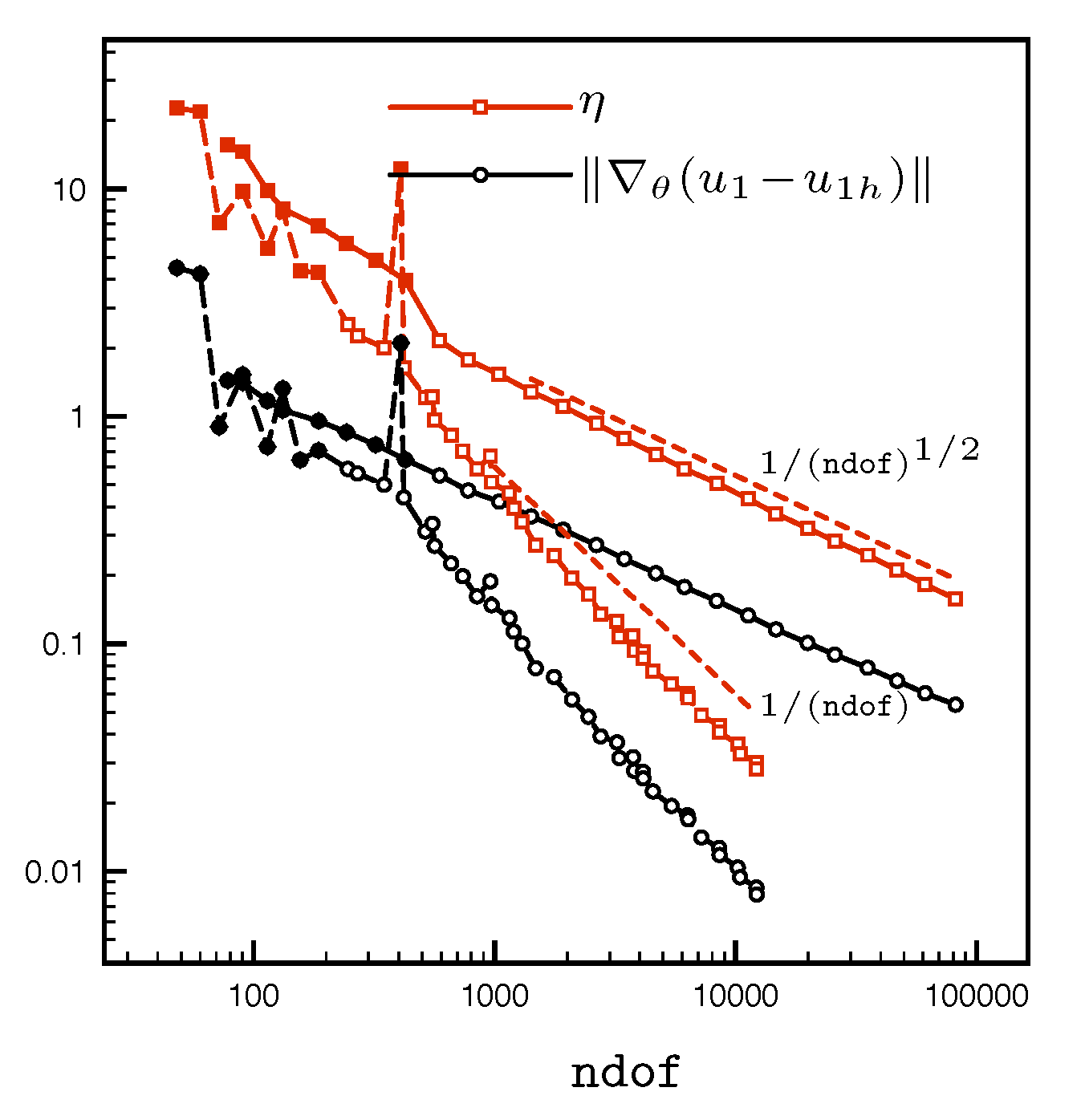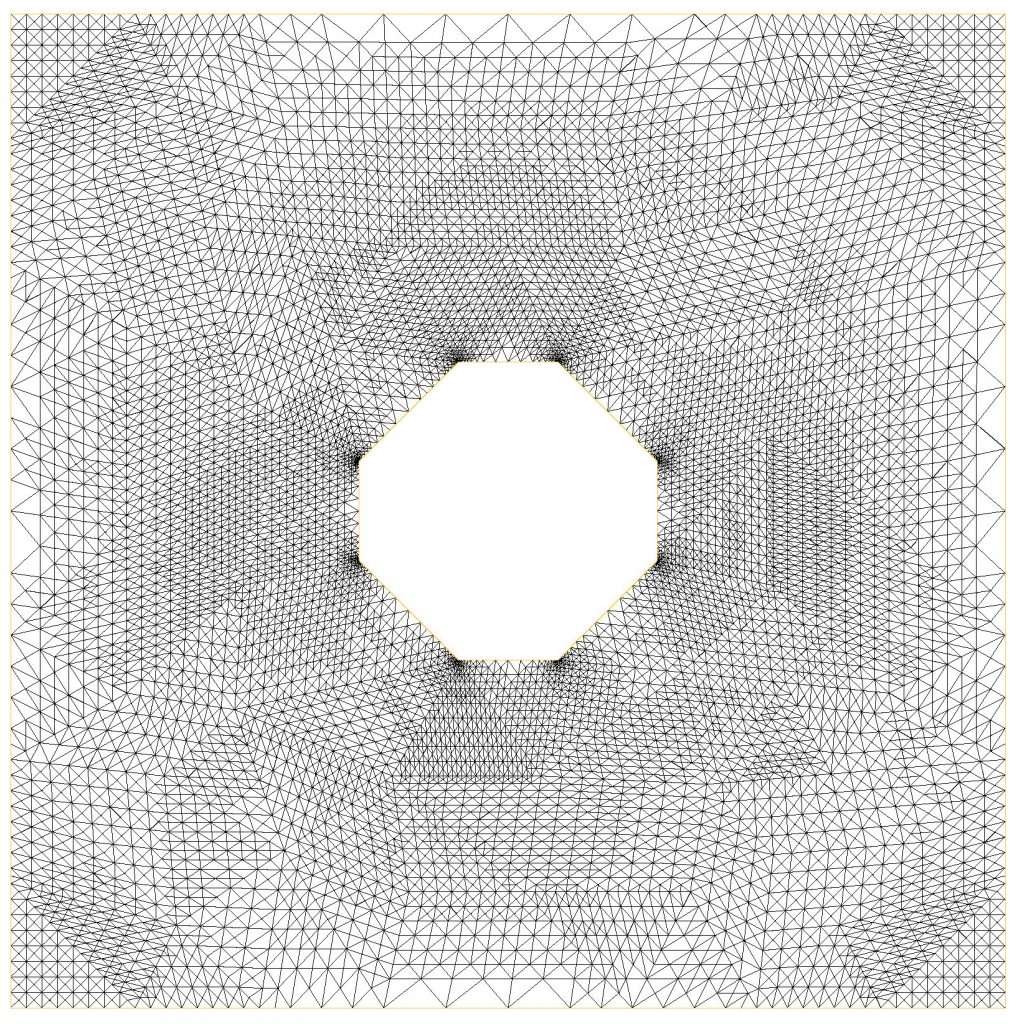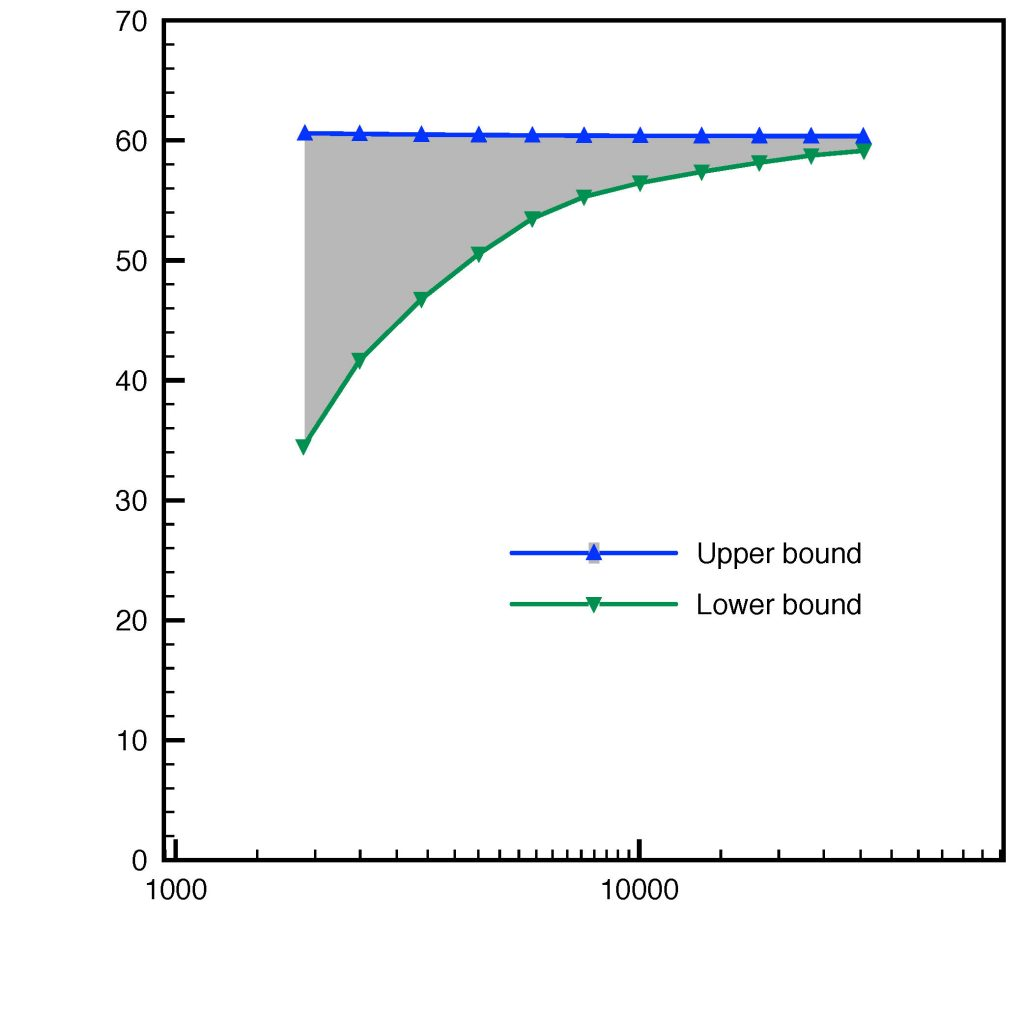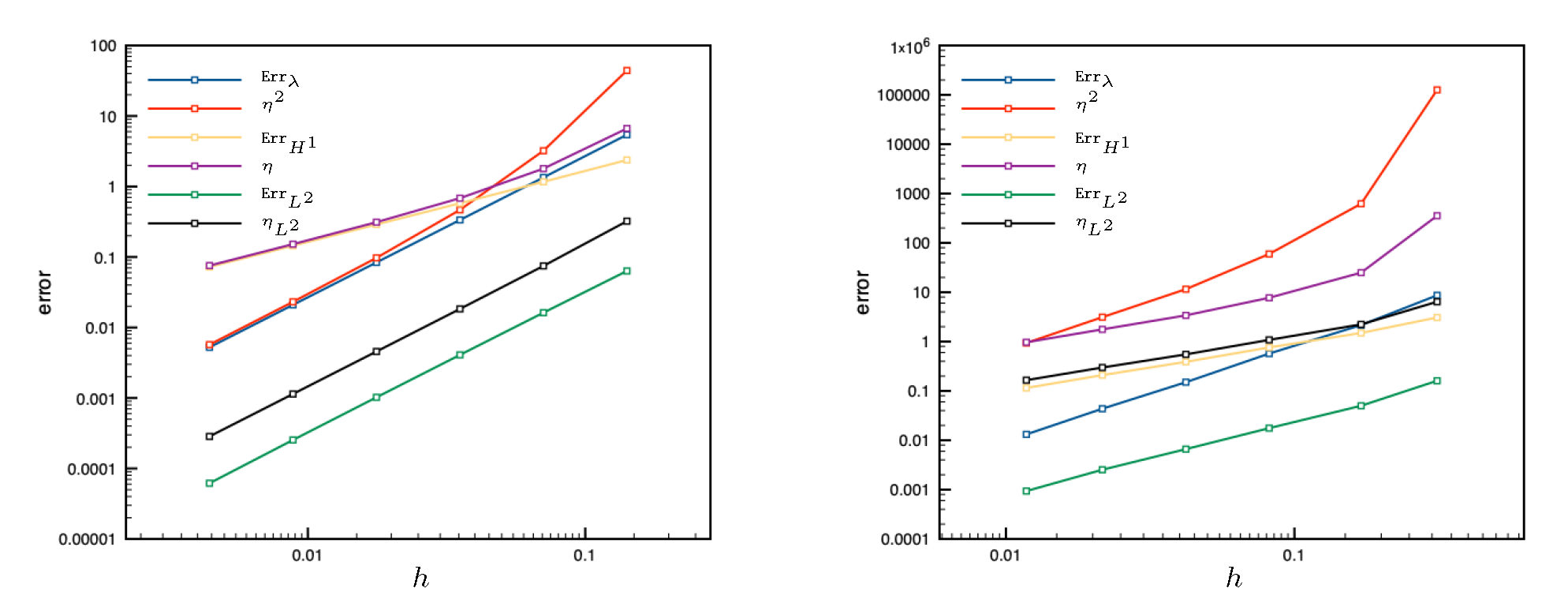# Guaranteed eigenvalue and eigenvector boundsFirst eigenvalue and eigenvector bounds on a square (structured mesh, 1st order conforming finite elements)First eigenvector bounds on an L-shaped domain (adaptively refined mesh, 1st and 2nd order discontinuous Galerkin elements)Adaptively refined mesh, domain with a holeGuaranteed inclusion of the first eigenvalue, domain with a holeEigenvalue and eigenvector bounds, 2-cluster on a square (left) and 3-cluster on an L-shaped domain (right), 1st order conforming finite elements

Main results:

• guaranteed upper and lower bounds for an arbitrary simple eigenvalue, multiple eigenvalue, or a cluster of eigenvalues;
• guaranteed energy error bounds on the associated eigenvector(s);
• efficiency (optimal convergence rate) and polynomial-degree robustness;
• multiplicative constant tending to one under an elliptic regularity assumption on the corresponding
source problem;
• inexact algebraic solvers taken into account;
• unified framework covering basically any numerical method (conforming, nonconforming, discontinuous Galerkin, and mixed finite elements);
• abstract framework for any second-order self-adjoint elliptic linear operator with compact resolvent.

Details: